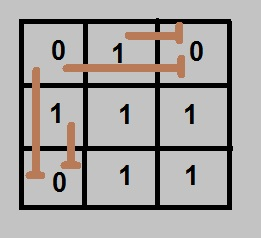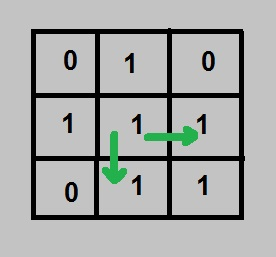Related Articles

# Find number of endless points

• Difficulty Level : Medium
• Last Updated : 10 Jun, 2021

Given a binary N x N matrix, we need to find the total number of matrix positions from which there is an endless path. Any position (i, j) is said to have an endless path if and only if the position (i, j) has the value 1 and all the next positions in its row(i) and its column(j) should have value 1. If any position next to (i, j) either in a row(i) or in column(j) will have 0 then position (i, j) doesn’t have any endless path.

Examples:

```Input :  0 1 0
1 1 1
0 1 1
Output : 4
Endless points are (1, 1), (1, 2),
(2, 1) and (2, 2). For all other
points path to some corner is
blocked at some point.

Input :  0 1 1
1 1 0
0 1 0
Output : 1
Endless point is (0, 1).```

Naive Approach :
We traverse all positions, for every position, we check that does this position has an endless path or not. If yes then count it otherwise ignore it. But as usual, its time complexity seems to be high.
Time complexity: O(n3)

We can easily say that if there is a zero at any position, then it will block the path for all the positions left to it and on top of it.Also, we can say that any position (i,j) will have an endless row if (i,j+1) will have an endless row and the value of (i, j) is 1.
Similarly, we can say that any position (i,j) will have an endless column if (i+1,j) will have an endless column and the value of (i, j) is 1.So we should maintain two matrices one for the row and one for the column. Always start from the rightmost position for row and bottom-most position for column and only check for next position whether it has an endless path or not.
And Finally, if any position will have an endless path in both row and column matrix then that position is said to have an endless path.

## C++

 `// C++ program to find count of endless points``#include``using` `namespace` `std;` `const` `int` `MAX = 100;` `// Returns count of endless points``int` `countEndless(``bool` `input[][MAX], ``int` `n)``{``    ``bool` `row[n][n], col[n][n];` `    ``// Fills column matrix. For every column, start``    ``// from every last row and fill every entry as``    ``// blockage after a 0 is found.``    ``for` `(``int` `j=0; j=0; i--)``        ``{``            ``// encountered a '0', set the isEndless``            ``// variable to false``            ``if` `(input[i][j] == 0)``                ``isEndless = 0;``            ``col[i][j] = isEndless;``        ``}``    ``}` `    ``// Similarly, fill row matrix``    ``for` `(``int` `i=0; i=0; j--)``        ``{``            ``if` `(input[i][j] == 0)``                ``isEndless = 0;``            ``row[i][j] = isEndless;``        ``}``    ``}` `    ``// Calculate total count of endless points``    ``int` `ans = 0;``    ``for` `(``int` `i=0; i

## Java

 `// Java program to find count of endless points``class` `GFG {``    ` `    ``static` `final` `int` `MAX = ``100``;``    ` `    ``// Returns count of endless points``    ``static` `int` `countEndless(``boolean` `input[][], ``int` `n)``    ``{``        ` `        ``boolean` `row[][] = ``new` `boolean``[n][n];``        ``boolean` `col[][] = ``new` `boolean``[n][n];``    ` `        ``// Fills column matrix. For every column,``        ``// start from every last row and fill every``        ``// entry as blockage after a 0 is found.``        ``for` `(``int` `j = ``0``; j < n; j++)``        ``{``            ` `            ``// flag which will be zero once we get``            ``// a '0' and it will be 1 otherwise``            ``boolean` `isEndless = ``true``;``            ``for` `(``int` `i = n-``1``; i >= ``0``; i--)``            ``{``                ` `                ``// encountered a '0', set the``                ``// isEndless variable to false``                ``if` `(input[i][j] == ``false``)``                    ``isEndless = ``false``;``                    ` `                ``col[i][j] = isEndless;``            ``}``        ``}``    ` `        ``// Similarly, fill row matrix``        ``for` `(``int` `i = ``0``; i < n; i++)``        ``{``            ``boolean` `isEndless = ``true``;``            ``for` `(``int` `j = n-``1``; j >= ``0``; j--)``            ``{``                ``if` `(input[i][j] == ``false``)``                    ``isEndless = ``false``;``                ``row[i][j] = isEndless;``            ``}``        ``}``    ` `        ``// Calculate total count of endless points``        ``int` `ans = ``0``;``        ``for` `(``int` `i = ``0``; i < n; i++)``            ``for` `(``int` `j = ``1``; j < n; j++)``    ` `                ``// If there is NO blockage in row``                ``// or column after this point,``                ``// increment result.``                ``if` `(row[i][j] && col[i][j])``                    ``ans++;``    ` `        ``return` `ans;``    ``}``    ` `    ``//driver code``    ``public` `static` `void` `main(String arg[])``    ``{``        ``boolean` `input[][] = {``                    ``{``true``, ``false``, ``true``, ``true``},``                    ``{``false``, ``true``, ``true``, ``true``},``                    ``{``true``, ``true``, ``true``, ``true``},``                    ``{``false``, ``true``, ``true``, ``false``}};``        ``int` `n = ``4``;``    ` `        ``System.out.print(countEndless(input, n));``    ``}``}` `// This code is contributed by Anant Agarwal.`

## Python3

 `# Python3 program to find count of``# endless points``import` `numpy as np` `# Returns count of endless points``def` `countEndless(input_mat, n) :` `    ``row ``=` `np.zeros((n, n))``    ``col ``=` `np.zeros((n, n))` `    ``# Fills column matrix. For every column,``    ``# start from every last row and fill``    ``# every entry as blockage after a 0 is found.``    ``for` `j ``in` `range``(n) :``        ` `        ``# flag which will be zero once we``        ``# get a '0' and it will be 1 otherwise``        ``isEndless ``=` `1``        ` `        ``for` `i ``in` `range``(n ``-` `1``, ``-``1``, ``-``1``) :``        ` `            ``# encountered a '0', set the``            ``# isEndless variable to false``            ``if` `(input_mat[i][j] ``=``=` `0``) :``                ``isEndless ``=` `0``            ` `            ``col[i][j] ``=` `isEndless``        ` `    ``# Similarly, fill row matrix``    ``for` `i ``in` `range``(n) :``        ` `        ``isEndless ``=` `1``        ``for` `j ``in` `range``(n ``-` `1``, ``-``1``, ``-``1``) :``            ` `            ``if` `(input_mat[i][j] ``=``=` `0``) :``                ``isEndless ``=` `0``                ` `            ``row[i][j] ``=` `isEndless``        ` `    ``# Calculate total count of endless points``    ``ans ``=` `0``    ``for` `i ``in` `range``(n) :``        ``for` `j ``in` `range``(``1``, n) :` `            ``# If there is NO blockage in row``            ``# or column after this point,``            ``# increment result.``            ``#print(row[i][j] , col[i][j])``            ``if` `(row[i][j] ``and` `col[i][j]) :``                ``ans ``+``=` `1``            ``#print(ans)` `    ``return` `ans` `# Driver code``if` `__name__ ``=``=` `"__main__"` `:` `    ``input_mat ``=` `[[``1``, ``0``, ``1``, ``1``],``                 ``[``0``, ``1``, ``1``, ``1``],``                 ``[``1``, ``1``, ``1``, ``1``],``                 ``[``0``, ``1``, ``1``, ``0``]]``    ``n ``=` `4` `    ``print``(countEndless(input_mat, n))``    ` `# This code is contributed by Ryuga`

## C#

 `// C# program to find count of``// endless points``using` `System;` `public` `class` `GFG {` `    ``// Returns count of endless points``    ``static` `int` `countEndless(``bool` `[,]input, ``int` `n)``    ``{``        ` `        ``bool` `[,]row = ``new` `bool``[n,n];``        ``bool` `[,]col = ``new` `bool``[n,n];``    ` `        ``// Fills column matrix. For every``        ``// column, start from every last``        ``// row and fill every entry as``        ``// blockage after a 0 is found.``        ``for` `(``int` `j = 0; j < n; j++)``        ``{``            ` `            ``// flag which will be zero``            ``// once we get a '0' and it``            ``// will be 1 otherwise``            ``bool` `isEndless = ``true``;``            ``for` `(``int` `i = n - 1; i >= 0; i--)``            ``{``                ` `                ``// encountered a '0', set``                ``// the isEndless variable``                ``// to false``                ``if` `(input[i,j] == ``false``)``                    ``isEndless = ``false``;``                    ` `                ``col[i,j] = isEndless;``            ``}``        ``}``    ` `        ``// Similarly, fill row matrix``        ``for` `(``int` `i = 0; i < n; i++)``        ``{``            ``bool` `isEndless = ``true``;``            ``for` `(``int` `j = n - 1; j >= 0; j--)``            ``{``                ``if` `(input[i,j] == ``false``)``                    ``isEndless = ``false``;``                ``row[i,j] = isEndless;``            ``}``        ``}``    ` `        ``// Calculate total count of``        ``// endless points``        ``int` `ans = 0;``        ``for` `(``int` `i = 0; i < n; i++)``            ``for` `(``int` `j = 1; j < n; j++)``    ` `                ``// If there is NO blockage``                ``// in row or column after``                ``// this point, increment``                ``// result.``                ``if` `(row[i,j] && col[i,j])``                    ``ans++;``    ` `        ``return` `ans;``    ``}``    ` `    ``//Driver code``    ``public` `static` `void` `Main()``    ``{``        ``bool` `[,]input = {``                ``{``true``, ``false``, ``true``, ``true``},``                ``{``false``, ``true``, ``true``, ``true``},``                ``{``true``, ``true``, ``true``, ``true``},``                ``{``false``, ``true``, ``true``, ``false``}};``        ``int` `n = 4;``    ` `        ``Console.Write(countEndless(input, n));``    ``}``}` `// This code is contributed by Sam007.`

## PHP

 `= 0; ``\$i``--)``        ``{``            ``// encountered a '0',``            ``// set the isEndless``            ``// variable to false``            ``if` `(``\$input``[``\$i``][``\$j``] == 0)``                ``\$isEndless` `= 0;``            ``\$col``[``\$i``][``\$j``] = ``\$isEndless``;``        ``}``    ``}` `    ``// Similarly, fill row matrix``    ``for` `(``\$i` `= 0; ``\$i` `< ``\$n``; ``\$i``++)``    ``{``        ``\$isEndless` `= 1;``        ``for` `(``\$j` `= ``\$n` `- 1; ``\$j` `>= 0; ``\$j``--)``        ``{``            ``if` `(``\$input``[``\$i``][``\$j``] == 0)``                ``\$isEndless` `= 0;``            ``\$row``[``\$i``][``\$j``] = ``\$isEndless``;``        ``}``    ``}` `    ``// Calculate total count``    ``// of endless points``    ``\$ans` `= 0;``    ``for` `(``\$i` `= 0; ``\$i` `< ``\$n``; ``\$i``++)``        ``for` `(``\$j` `= 1; ``\$j` `< ``\$n``; ``\$j``++)` `            ``// If there is NO blockage``            ``// or column after this point,``            ``// increment result.``            ``if` `(``\$row``[``\$i``][``\$j``] &&``                ``\$col``[``\$i``][``\$j``])``                ``\$ans``++;` `    ``return` `\$ans``;``}` `// Driver code``\$input` `= ``array``(``array``(1, 0, 1, 1),``               ``array``(0, 1, 1, 1),``               ``array``(1, 1, 1, 1),``               ``array``(0, 1, 1, 0));``\$n` `= 4;` `echo` `countEndless(``\$input``, ``\$n``);` `// This code is contributed``// by shiv_bhakt.``?>`

## Javascript

 ``

Output:

`5`